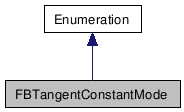# FBTangentConstantMode Class Reference

FBTangentConstantMode FBTangentConstantMode FBTangentConstantMode FBTangentConstantMode FBTangentConstantMode FBTangentConstantMode FBTangentConstantMode FBTangentConstantMode FBTangentConstantMode FBTangentConstantMode FBTangentConstantMode FBTangentConstantMode FBTangentConstantMode FBTangentConstantMode FBTangentConstantMode FBTangentConstantMode FBTangentConstantMode FBTangentConstantMode FBTangentConstantMode FBTangentConstantMode FBTangentConstantMode FBTangentConstantMode FBTangentConstantMode FBTangentConstantMode FBTangentConstantMode FBTangentConstantMode FBTangentConstantMode FBTangentConstantMode FBTangentConstantMode FBTangentConstantMode FBTangentConstantMode FBTangentConstantMode FBTangentConstantMode FBTangentConstantMode FBTangentConstantMode FBTangentConstantMode FBTangentConstantMode FBTangentConstantMode FBTangentConstantMode FBTangentConstantMode FBTangentConstantMode FBTangentConstantMode FBTangentConstantMode FBTangentConstantMode FBTangentConstantMode FBTangentConstantMode FBTangentConstantMode FBTangentConstantMode FBTangentConstantMode FBTangentConstantMode FBTangentConstantMode FBTangentConstantMode FBTangentConstantMode FBTangentConstantMode FBTangentConstantMode FBTangentConstantMode FBTangentConstantMode FBTangentConstantMode FBTangentConstantMode FBTangentConstantMode FBTangentConstantMode FBTangentConstantMode FBTangentConstantMode FBTangentConstantMode FBTangentConstantMode FBTangentConstantMode FBTangentConstantMode FBTangentConstantMode FBTangentConstantMode FBTangentConstantMode FBTangentConstantMode FBTangentConstantMode FBTangentConstantMode FBTangentConstantMode FBTangentConstantMode FBTangentConstantMode FBTangentConstantMode FBTangentConstantMode FBTangentConstantMode FBTangentConstantMode FBTangentConstantMode FBTangentConstantMode FBTangentConstantMode FBTangentConstantMode FBTangentConstantMode FBTangentConstantMode FBTangentConstantMode FBTangentConstantMode FBTangentConstantMode FBTangentConstantMode FBTangentConstantMode FBTangentConstantMode FBTangentConstantMode FBTangentConstantMode FBTangentConstantMode FBTangentConstantMode FBTangentConstantMode FBTangentConstantMode FBTangentConstantMode FBTangentConstantMode FBTangentConstantMode FBTangentConstantMode FBTangentConstantMode FBTangentConstantMode FBTangentConstantMode FBTangentConstantMode FBTangentConstantMode FBTangentConstantMode FBTangentConstantMode FBTangentConstantMode FBTangentConstantMode FBTangentConstantMode FBTangentConstantMode FBTangentConstantMode FBTangentConstantMode FBTangentConstantMode FBTangentConstantMode FBTangentConstantMode FBTangentConstantMode FBTangentConstantMode
Inheritance diagram for FBTangentConstantMode:[legend]

List of all members.

## Detailed Description

Different constant modes for the tangents.

## Public Attributes

kFBTangentConstantModeNormal
The tangent will contain the value of the current keyframe until the next keyframe.
kFBTangentConstantModeNext
The tangent will contain the value of the next keyframe.

## Member Data Documentation

The tangent will contain the value of the current keyframe until the next keyframe.

The tangent will contain the value of the next keyframe.

Generated on Tue Feb 9 19:34:40 2010 for Python Reference Guide by1.5.3